Name:    3rd Grade Number & Operations—FractionsDevelop understanding of fractions as numbers.  Standard 1  & 2, Test 1

Multiple Choice
Identify the choice that best completes the statement or answers the question.

1.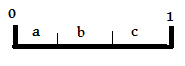Line 0 - 1 is a whole line.  What fraction of line 0 - 1 does section “a” represent?
 a.c.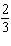b.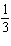d. 1

2.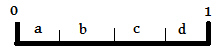Line 0 - 1 is a whole line.  What fraction of line 0 - 1 do sections “c and d” represent?
 a. 1 c. 2 b.d.3.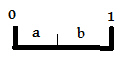Line 0 - 1 is a whole line.  What fraction of line 0 - 1 do sections “a and b” represent?
 a. 1 c. 2 b.d.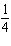4.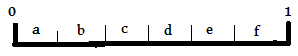Line 0 - 1 is a whole line.  What fraction of line 0 - 1 do sections “a, b, c, d,” represent?
 a.c.b. 1 d.5.What fraction of the whole line do letters e, f, g, h represent?
 a.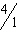c.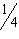b.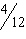d.6.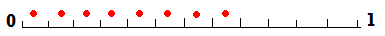What fraction of the whole line has red dots?
 a.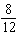c.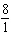b.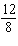d. 1

7.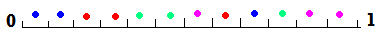What fraction of the whole line has colored dots?
 a.c.b.d.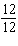8.What fraction of the whole line has blue and green colored dots?
 a.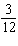c.b.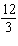d.9.Which letter can be found at 1/12 of the whole line?
 a. A c. E b. B d. D

10.Which letter can be found at 7/12 of the whole line?
 a. A c. E b. B d. C